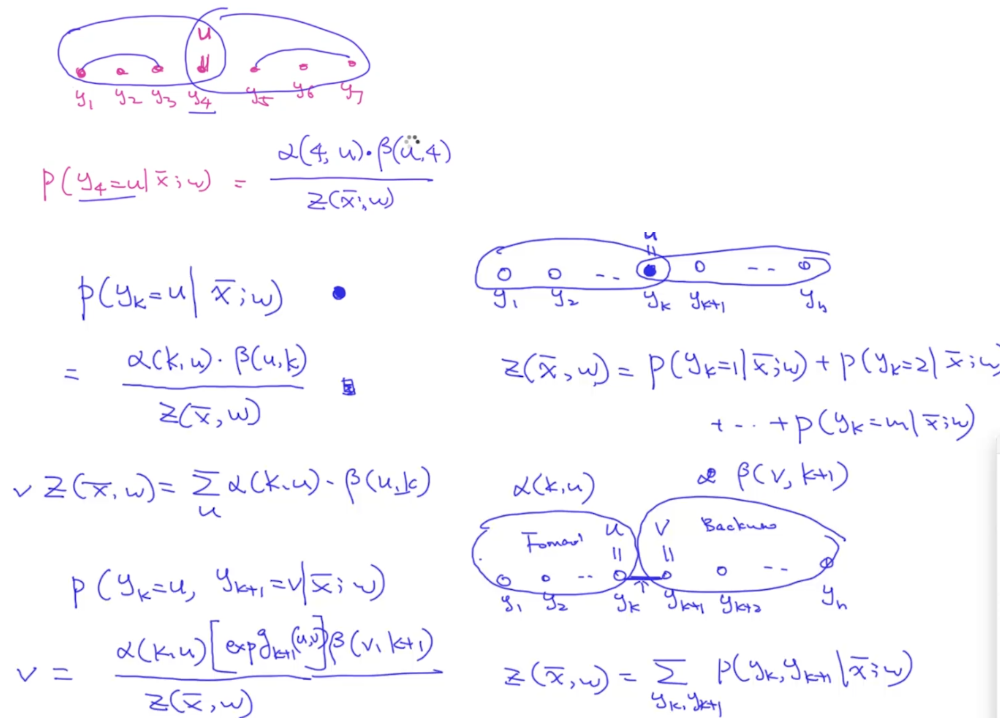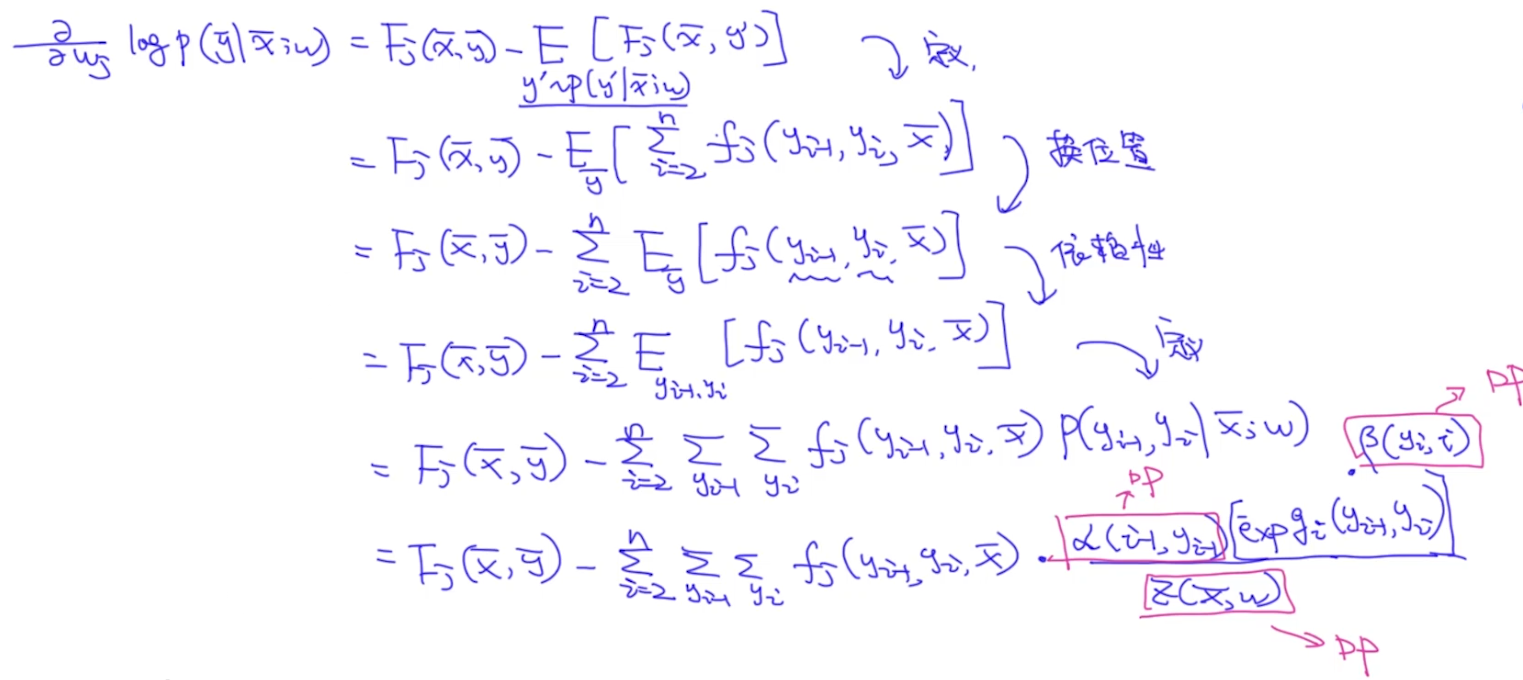# CRF学习——基础学习

CRF是NLP中很常用且经典的模型，今天就复习一下CRF模型。

# 前言

## 生成模型 VS 判别模型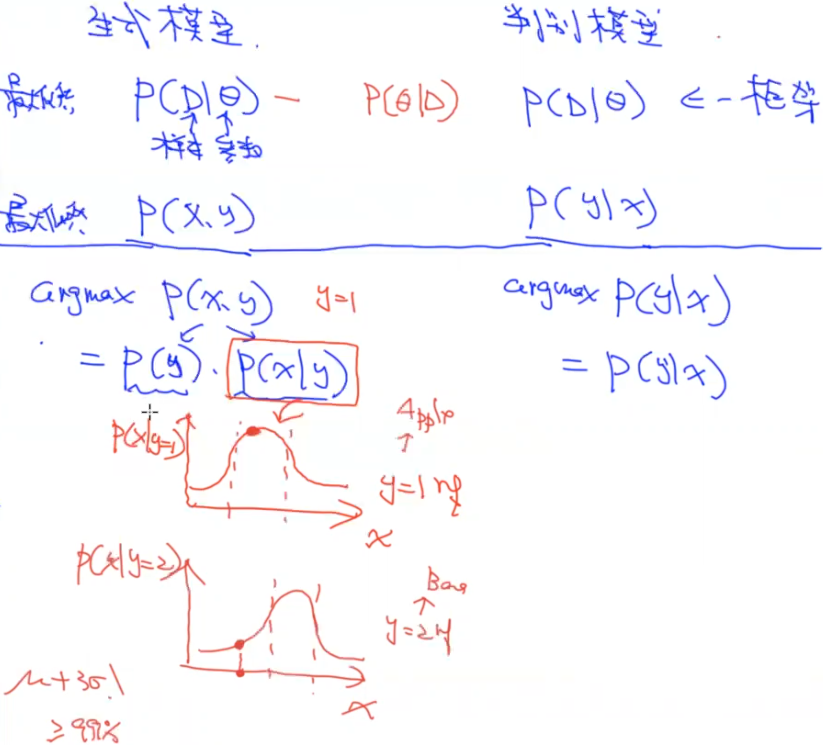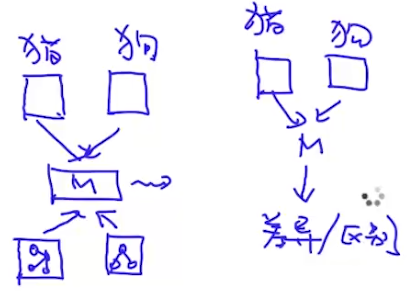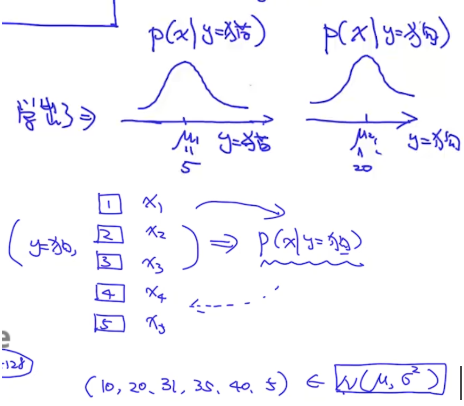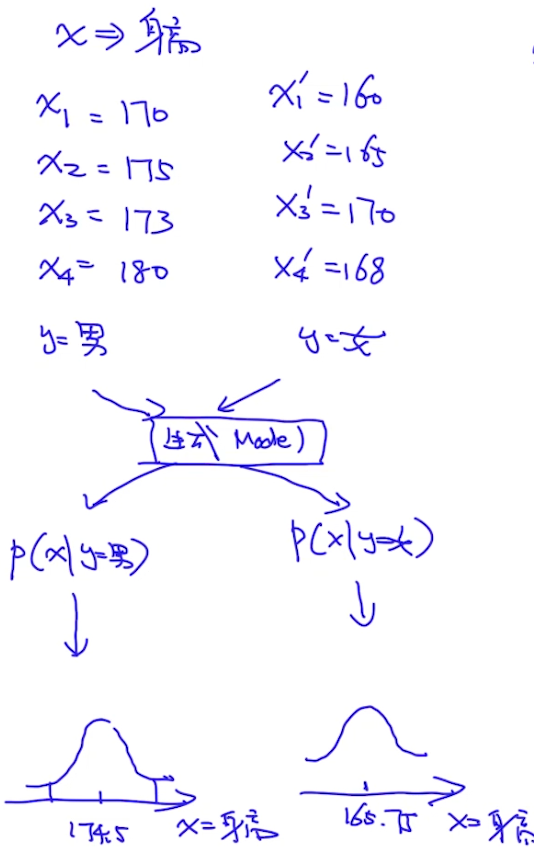• 当数据量大时，使用判别模型会优于生成模型
• 当数据量小时，生成模型有可能比判别模型要好（因为生成模型有prior，即p(x)，有时为了防止过拟合，会在模型中加入正则项，其实这个正则项就是一个prior）

【注】：上面提到的p(x, y)、p(x|y)其实是所有概率的乘积，即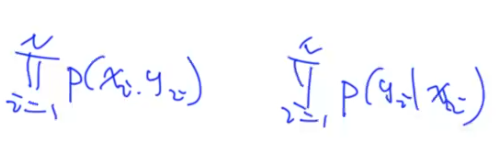## Directed Model VS Undirected Model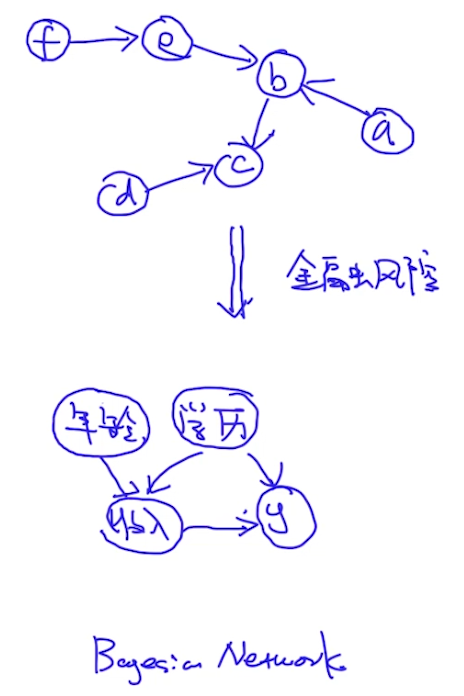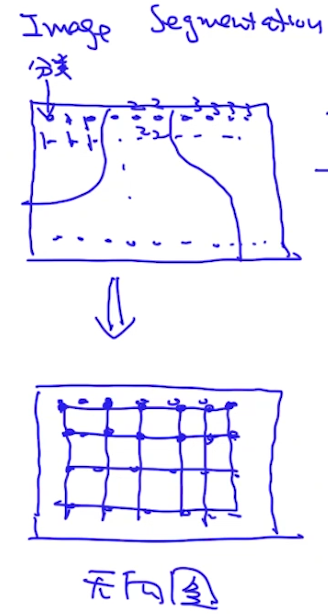## Joint Probability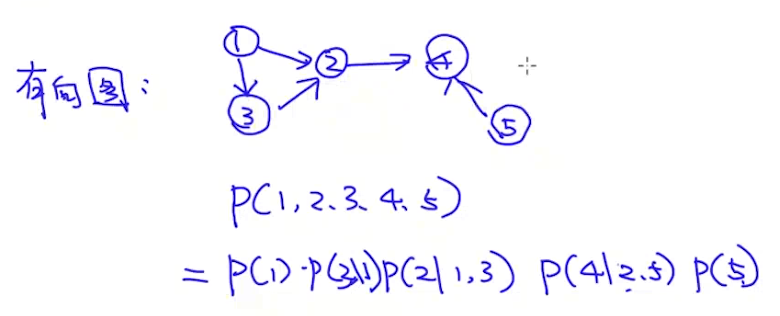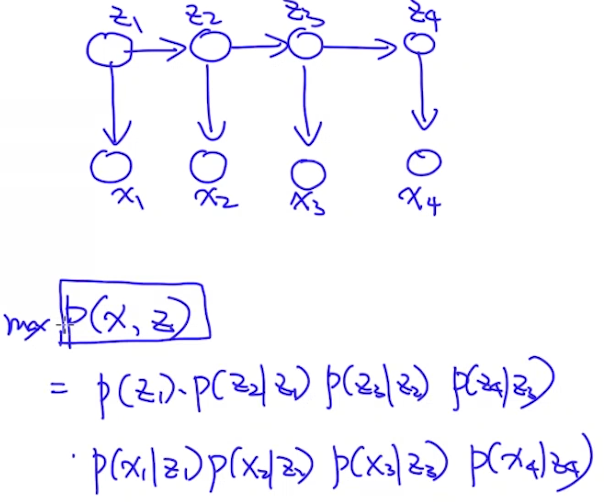• Maximal Clique方法：先分成团，每个团用score function计算一个契合度。而这个契合度和团里的元素的联合概率是正相关的，因此这个score function也可以用联合概率表示，如下图：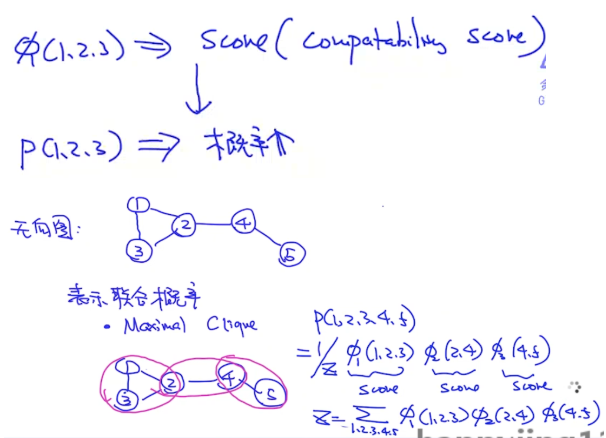• Pair-wise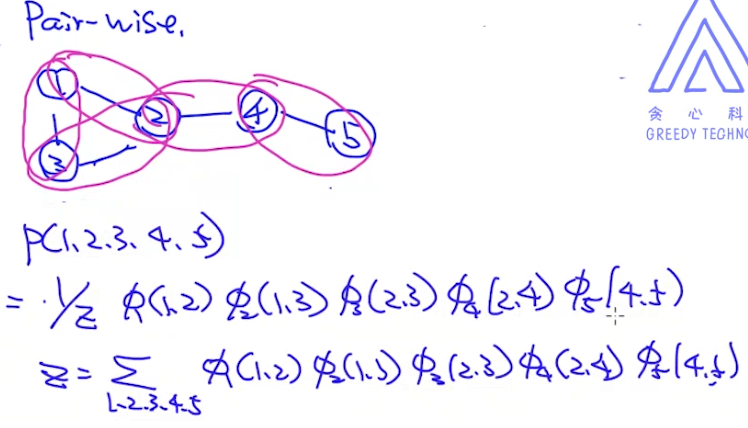【注】：

• 因为score function得出的是一个相对值，不同feature、不同算法得出的结果不一样。但最后我们是想得到P(1,2,3,4,5)这个联合概率的绝对值的（后续做判别时会用到），因此我们除以了一个归一化项z。这个z要考虑所有可能的情况，在实际情况中我们可以使用维特比来简化计算量。
• z是一个global normalization，使用这种全局的归一化有一个好处，就是图中的结点是有一个偏好的，但是z是一个全局的量，可以修正结点的偏好，使得模型考虑得更加全面。
• 在有向图中使用的是local normalization，比如P(4|2,5)只需要关注P(2,5)，因此有向图是从局部角度考虑的
• z的计算量比较大，如果变量是离散型的，则可以使用维特比算法；如果变量是连续型的，则只能使用一些近似算法（partition funciton，比如MCMC）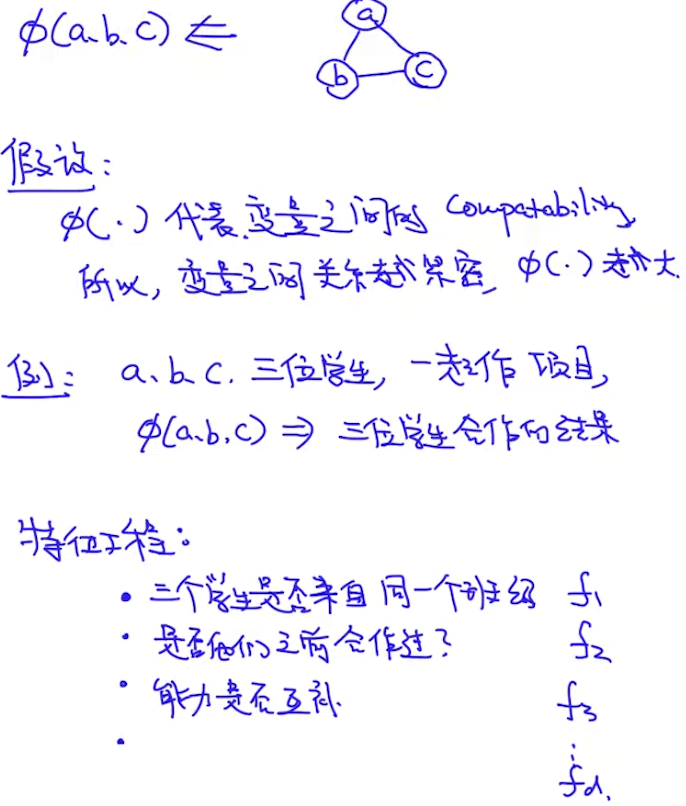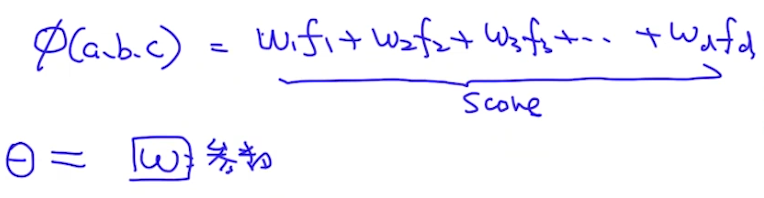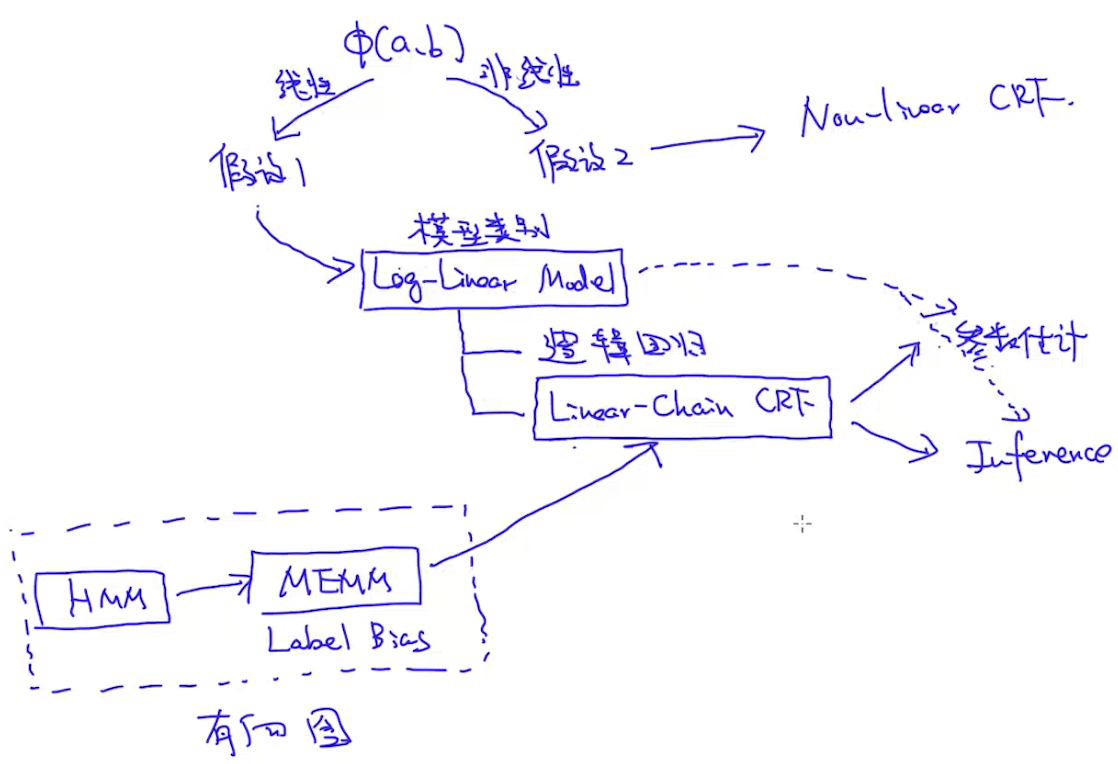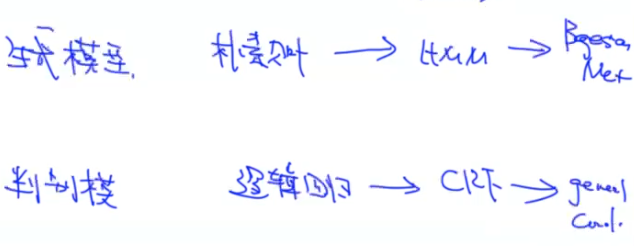# Log-Linear Model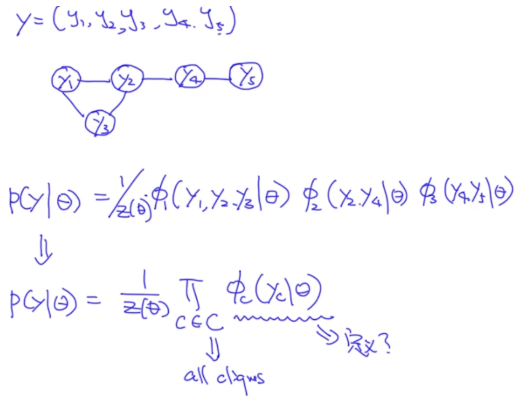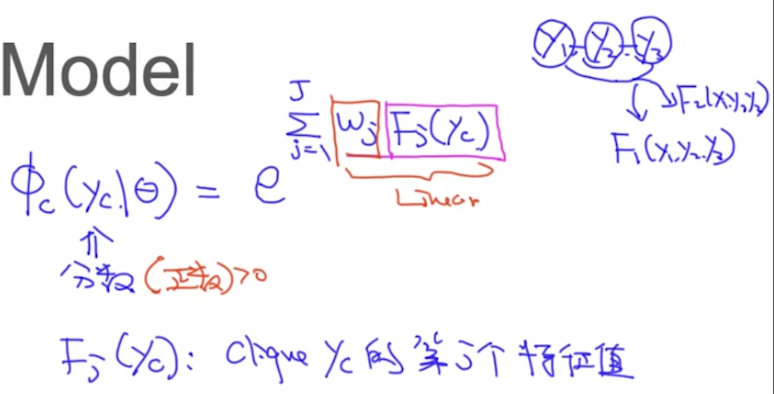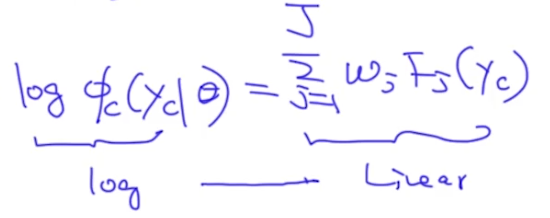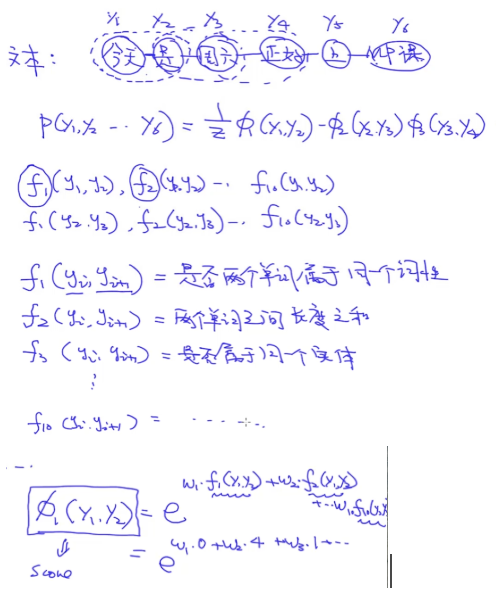## Multinomial Logistic Regression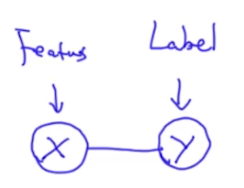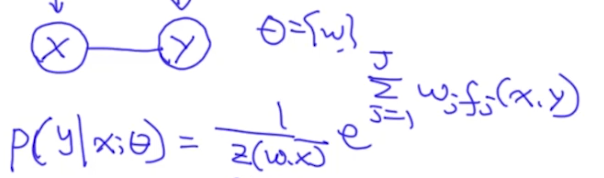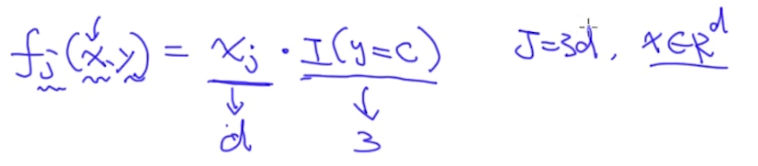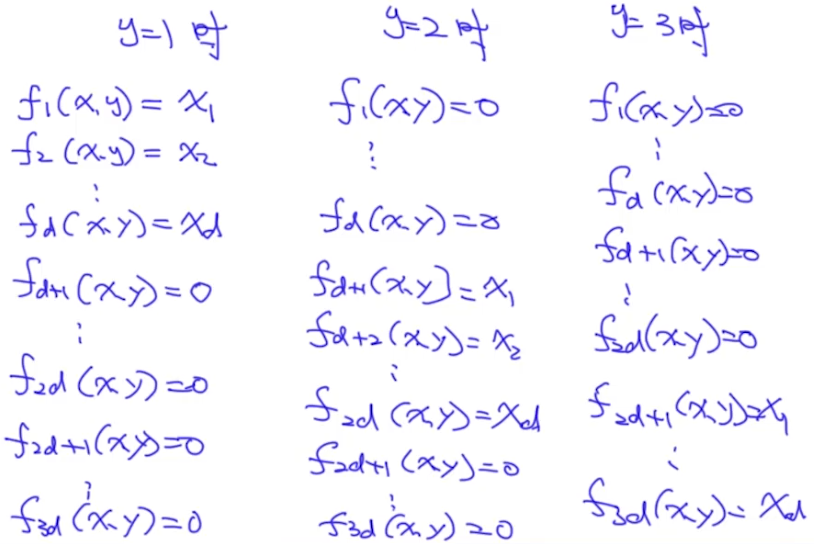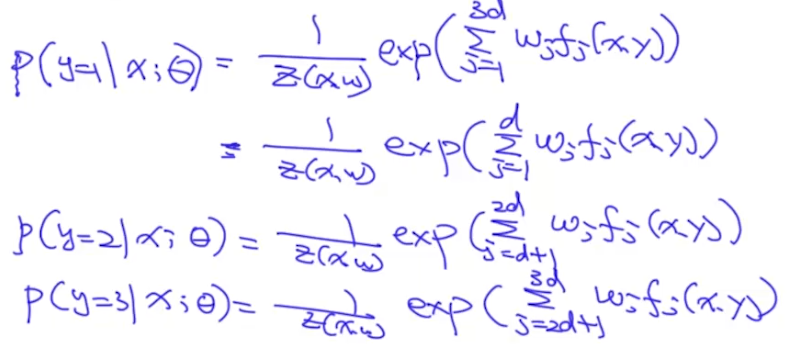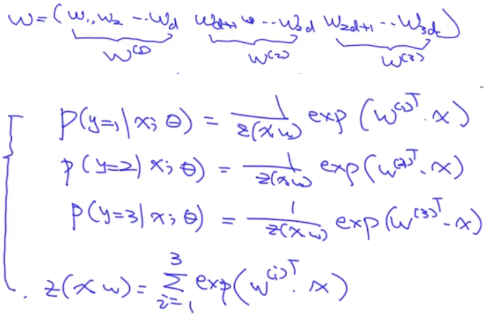# HMM存在的一些问题

### MEMM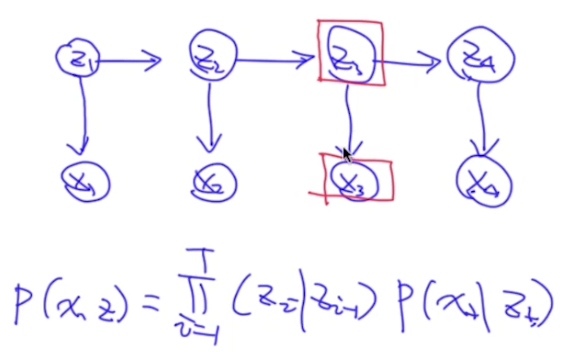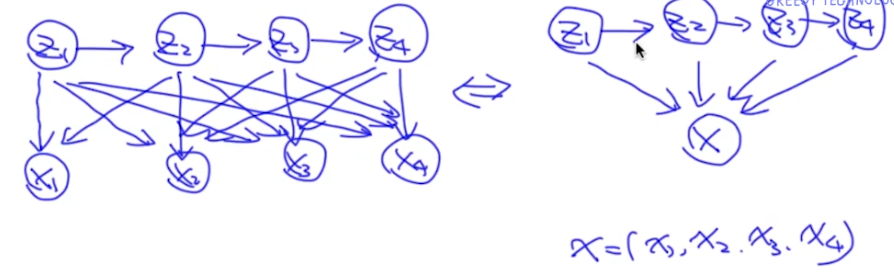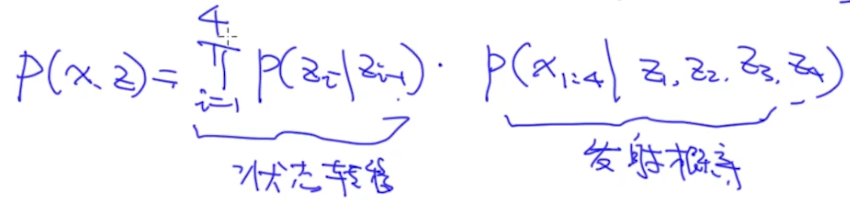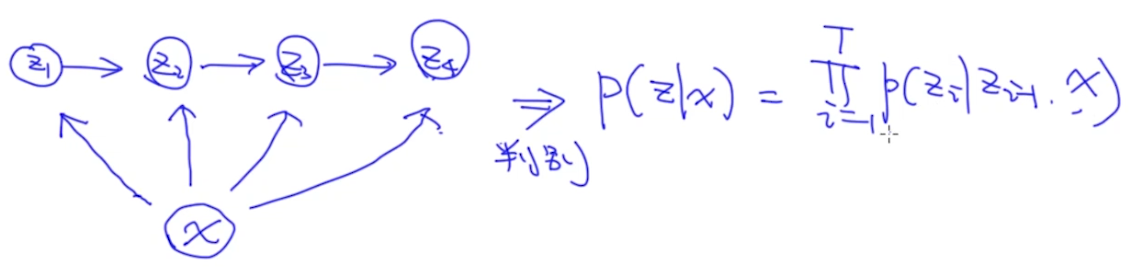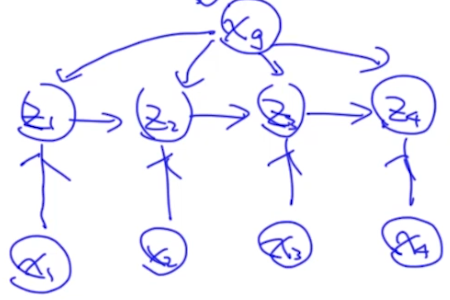### Label Bias Problem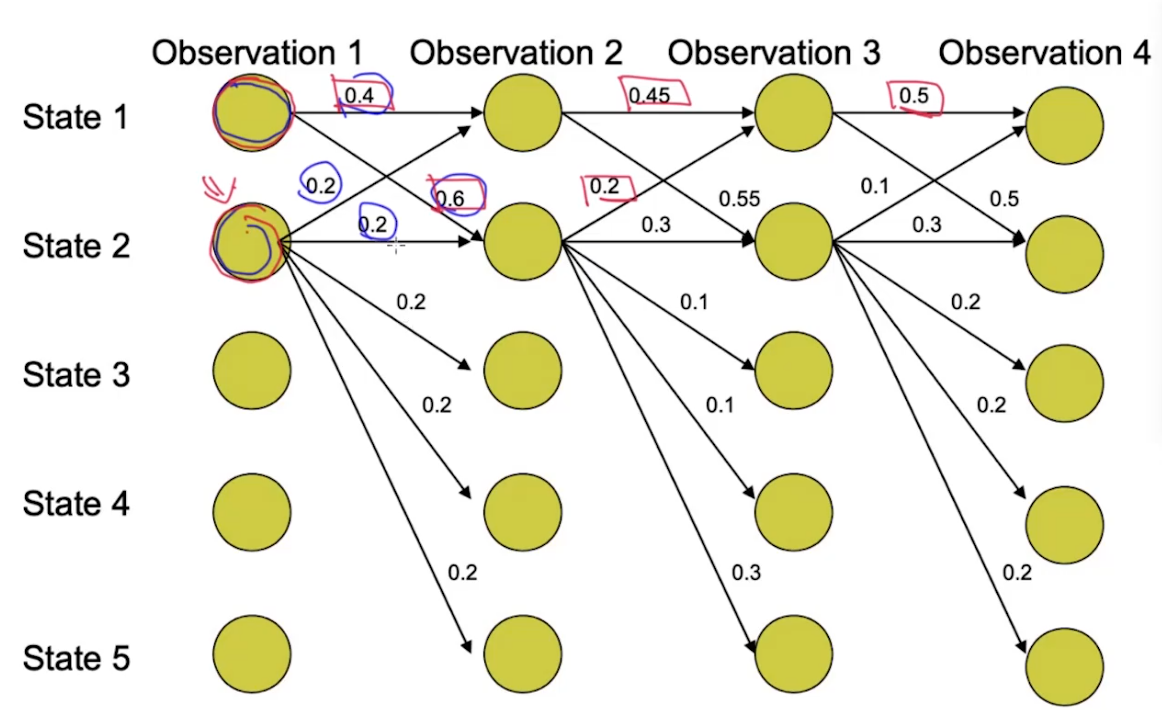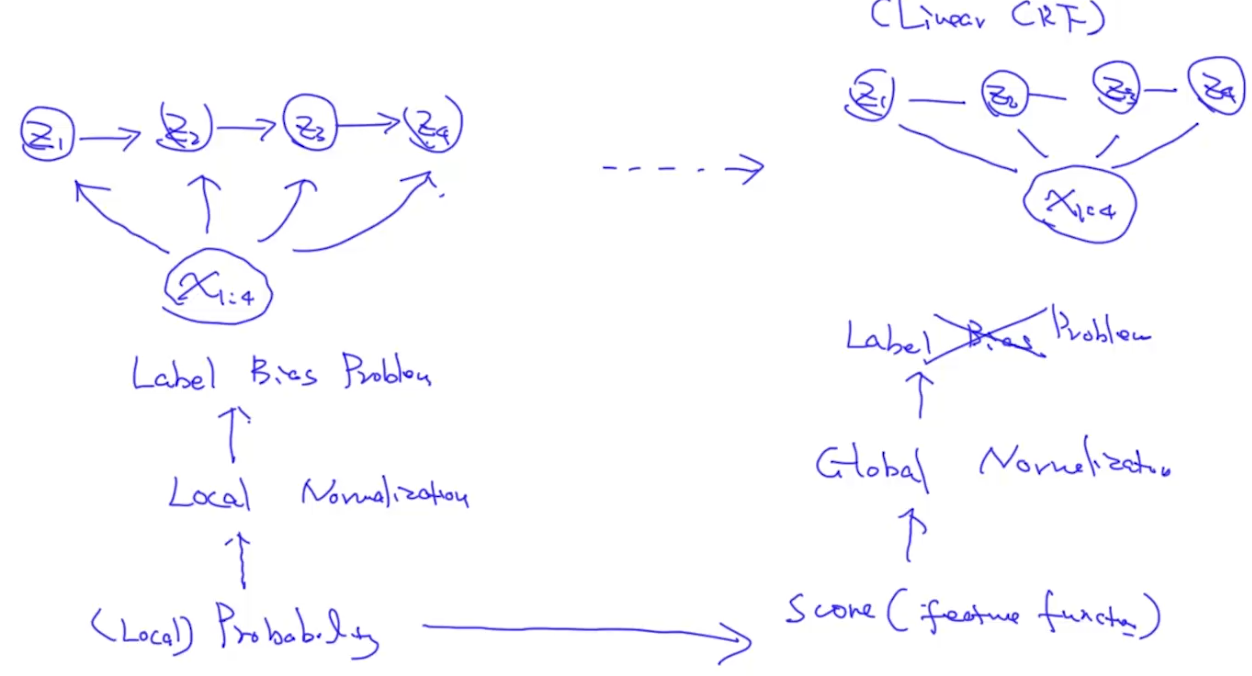### 从Log-linear Model到Log-linear CRF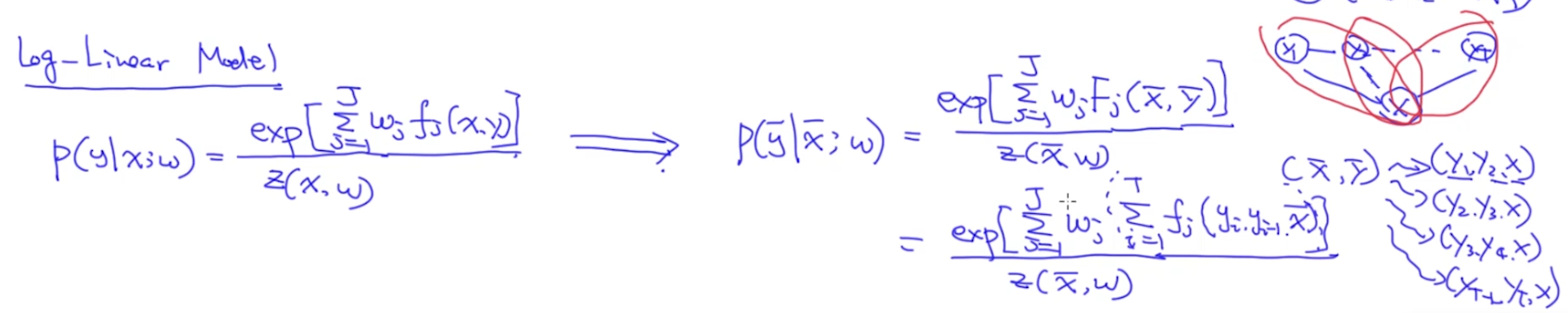$f_j$可以通过人工，或者神经网络的方式得到，接下来就是求解$w_j$。我们的目标是最大化似然函数：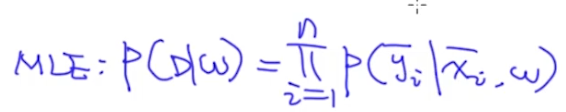，因此选择好一个优化方法，就可以求解$w_j$了（比如将loss作为分子对w求偏导）。

【注】：

• 上式中T是团的个数，在实践序列中，就是timestep-1
• 上式中exp有两个sum，其中第一个sum是对每个clique的结果求和（因为我们要把所有clique的结果乘起来），第二个sum是对每个clique内部所有特征的加和。？好像写反了
• $f_j(y_i, y_{i-1}, x)$中的$f_j$在神经网络中常使用softmax
• $f_j(y_i, y_{i-1}, x)$中的的x可以理解为x1, x2,…，即特征是根据$y_i, y_{i-1}$和所有的x计算出来的，即$f_j(y_i, y_{i-1}, x_1, x_2, …)$

# Log-linear CRF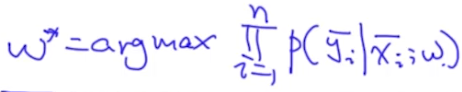## Inference Probelm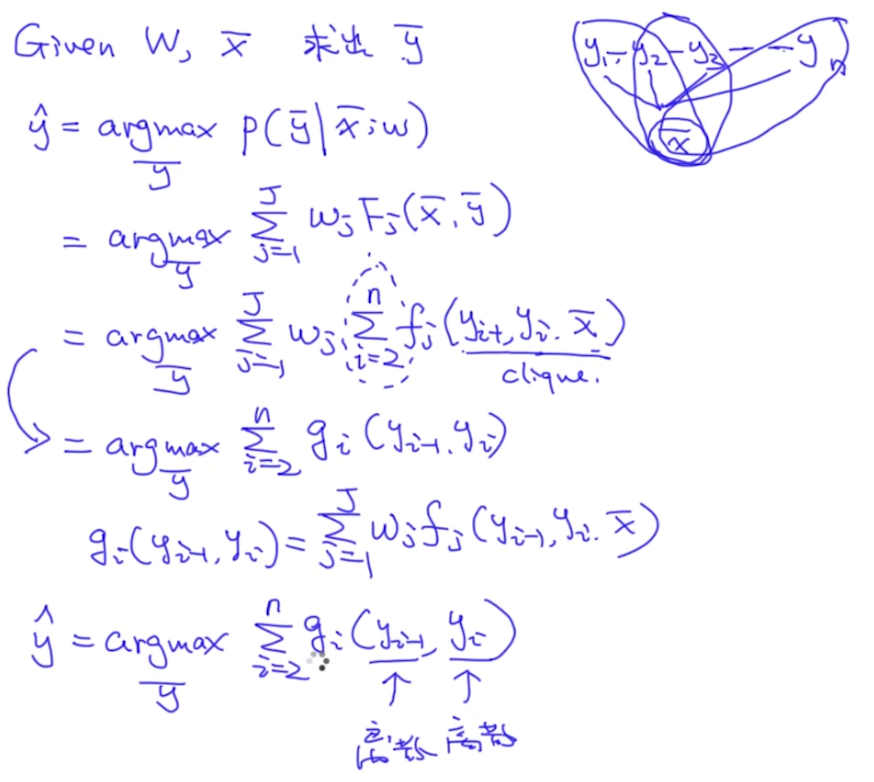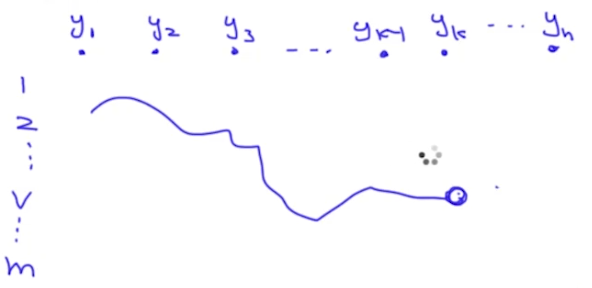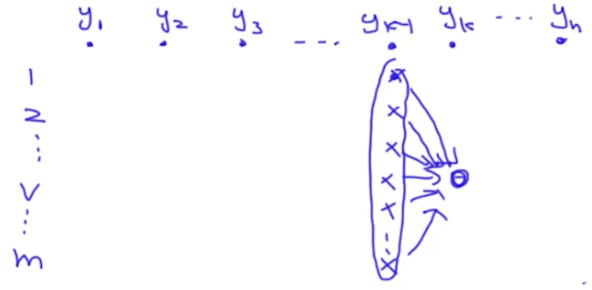【注】：如果score函数是$g_i(y_{i-2}, y_{i-1}, y_{i})$，那么算法复杂度就可能变为$O(m^2n)O(m)$

## Parameter Estimation

### Log-Linear的w求解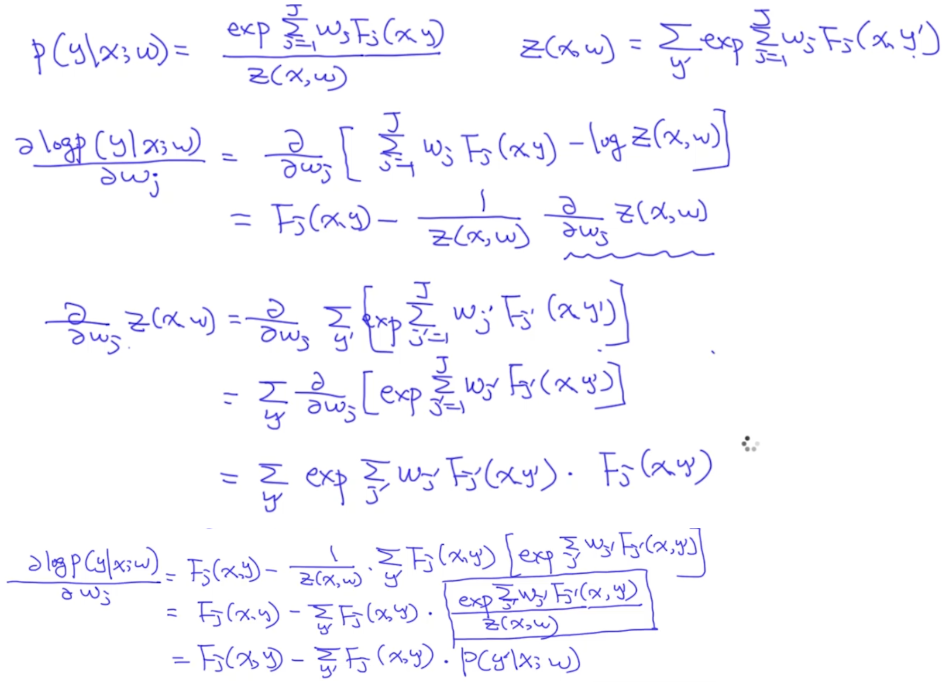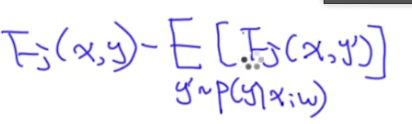### Log-Linear CRF的w求解

• 求解partition function z
• Forward算法：在CRF中，partition function z(x, w)中的x变成了一个序列，我们来看一下这个z怎么计算。我们依然可以使用动态规划算法求解$sum(g_i(y_{i-1}, y_i))$。具体如下图所示：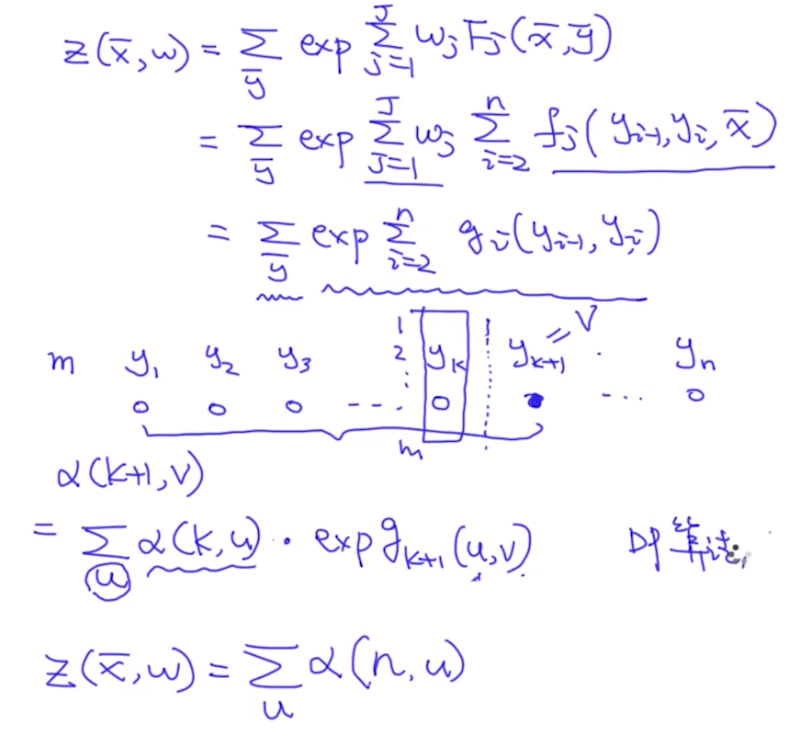• Backward算法：跟Forward相似，只不过是$y_k=u$时考虑${y_k{k+1}, y_n}$的所有可能性，这时候$y_{k+1}$成为了中介。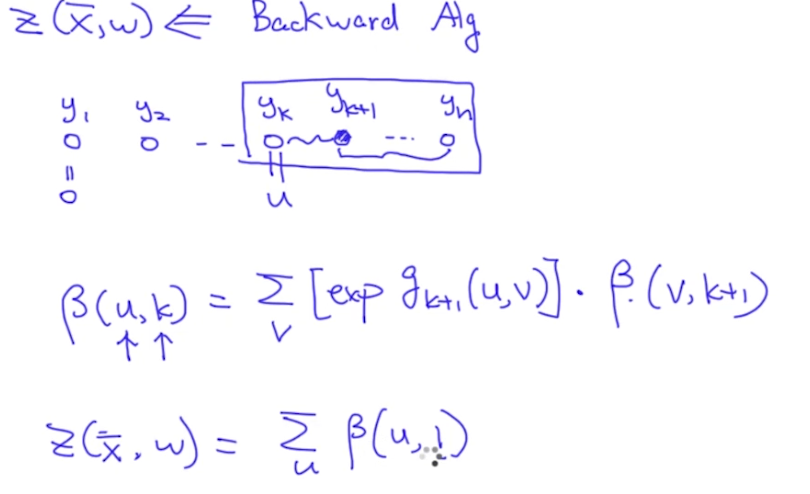• 求解$P(y_k=u|x;w)$

• $z(x, w)$是考虑所有的可能性，因此可以认为$z(x, w)=p(y_k=1|x;w) + p(y_k=2|x, w) + … + p(y_k=m|x;w)$
• 根据前向算法和后向算法，可以算出$z(x, w)$
• $p(y_k=u|x;w)$表示$y_k=u$的情况下，前向和后向的所有可能情况，如下图：